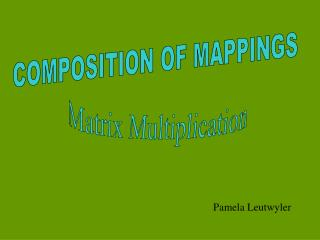# COMPOSITION OF MAPPINGS - PowerPoint PPT PresentationDownload PresentationCOMPOSITION OF MAPPINGS

COMPOSITION OF MAPPINGS
Download Presentation## COMPOSITION OF MAPPINGS

- - - - - - - - - - - - - - - - - - - - - - - - - - - E N D - - - - - - - - - - - - - - - - - - - - - - - - - - -
##### Presentation Transcript

1. COMPOSITION OF MAPPINGS Matrix Multiplication Pamela Leutwyler

2. = the matrix for T

3. = the matrix for S

4. R3 R2 T S R2 ST

5. R3 Find the matrix for ST R2 T S R2 ST

6. The first column of ST is S(the first column of T)

7. The second column of ST is S(the second column of T)

8. One way to define the product of two Matrices A and B is: THE nth COLUMN OF AB IS A( nth COLUMN OF B )

9. Because the matrix product AB represents a COMPOSITION OF MAPPINGS, It is important that the RANGE OF B is within The DOMAIN OF A. If A is an mn matrix, It has m rows and n columns. Its domain is Rn, and its range is Rm If B is an np matrix, It has n rows and p columns. Its domain is Rp, and its range is Rn We can form the product AB And the answer will be An mp matrix. mn np

10. Conformable: can be multiplied Consider the example: C D CD 32 24 34

11. Consider the example: C D CD 32 24 34

12. Consider the example: C D CD 32 24 34 To find the third column of CD take C times the third column of D

13. is row 2 of C DOT column 3 of D Consider the example: C D CD 1 32 24 34 To break this down further, consider the second entry in column 3 the entry in row 2 column 3 of CD

14. In general, for any two conformable matrices A and B: The entry in row j, column k of AB Is (row j of A) dot (column k of B) column k row j A B AB

15. Consider the example: C D CD

16. Consider the example: C D CD

17. Consider the example: C D CD

18. Consider the example: C D CD

19. Consider the example: C D CD

20. Consider the example: C D CD

21. Consider the example: C D CD Sorry – I skipped a few steps!

22. Consider the example: C D CD